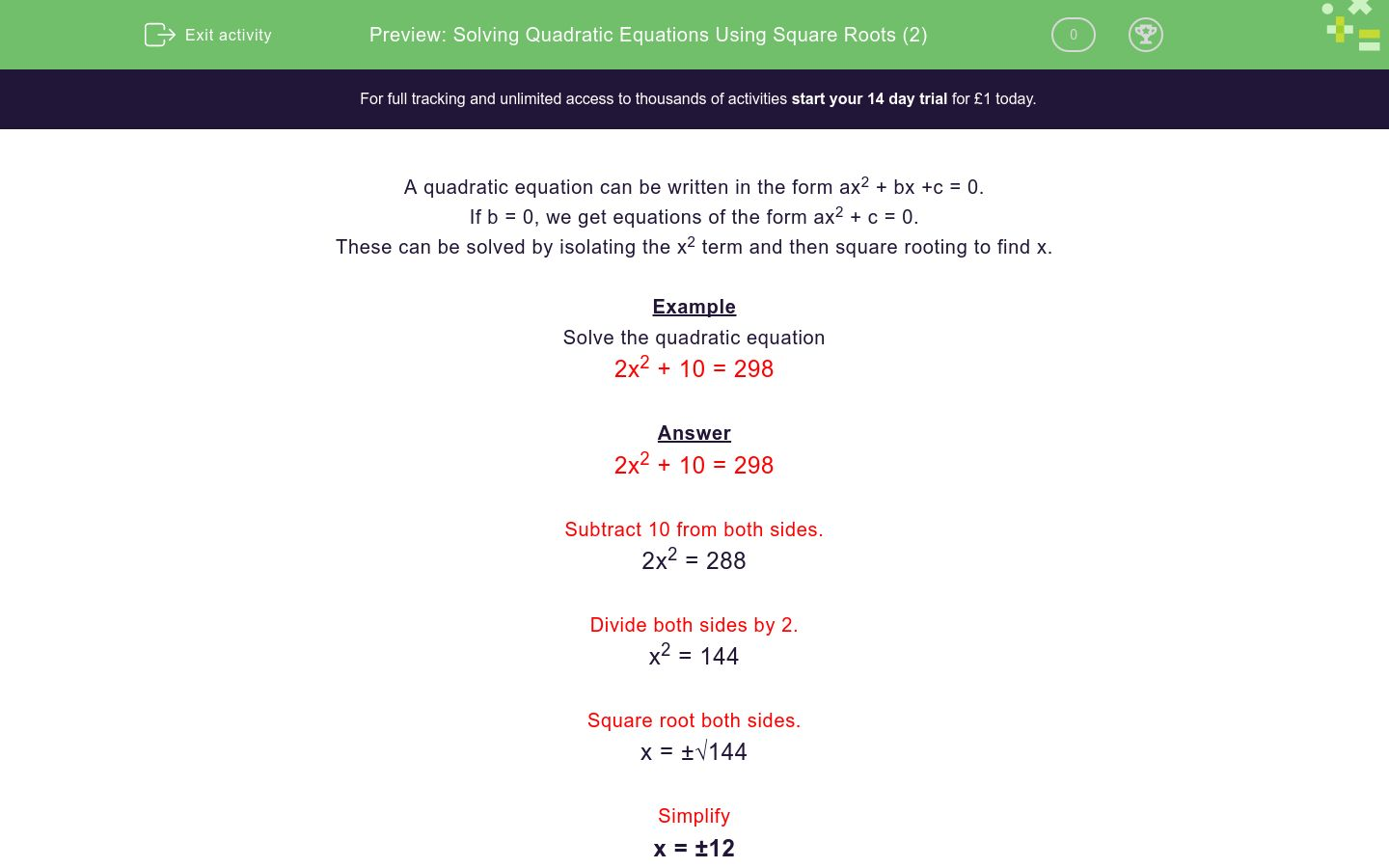# Solving Quadratic Equations Using Square Roots (2)

In this worksheet, students solve simple quadratic equations where there is just a squared term and a number.Key stage:  KS 4

Curriculum topic:  Algebra

Curriculum subtopic:  Solve Quadratic Equations by Factorising

Difficulty level:### QUESTION 1 of 10

A quadratic equation can be written in the form ax2 + bx +c = 0.

If b = 0, we get equations of the form ax2 + c = 0.

These can be solved by isolating the x2 term and then square rooting to find x.

Example

2x2 + 10 = 298

2x2 + 10 = 298

Subtract 10 from both sides.

2x2 = 288

Divide both sides by 2.

x2 = 144

Square root both sides.

x = ±√144

Simplify

x = ±12

Solve the quadratic equation by selecting all the correct solutions below.

x2 + 2 = 38

x = 6

x = 18

x = -6

x = -18

Solve the quadratic equation by selecting all the correct solutions below.

x2 - 7 = 9

x = -4

x = -3

x = 3

x = 4

Solve the quadratic equation by selecting all the correct solutions below.

x2 - 49 = 0

x = -7

x = 7

x = 4

x = -4

Solve the quadratic equation by selecting all the correct solutions below.

x2 - 1 = 0

x = -1

x = ½

x = 1

x = 0

Solve the quadratic equation by selecting all the correct solutions below.

x2 + 8 = 8

x = -1

x = -8;

x = 1

x = 0

Solve the quadratic equation by selecting all the correct solutions below.

2x2 + 6 = 24

x = -3

x = -9

x = 9

x = 3

Solve the quadratic equation by selecting all the correct solutions below.

2x2 -14 = 36

x = -6

x = -5

x = 5

x = 6

Solve the quadratic equation by selecting all the correct solutions below.

4x2 + 6 = 42

x = -9

x = 9

x = 3

x = -3

Solve the quadratic equation by selecting all the correct solutions below.

6x2 + 4 = 100

x = -3

x = 4

x = 3

x = -4

Solve the quadratic equation by selecting all the correct solutions below.

10x2 - 100 = 900

x = -100

x = -10

x = 10

x = 100

• Question 1

Solve the quadratic equation by selecting all the correct solutions below.

x2 + 2 = 38

x = 6
x = -6
• Question 2

Solve the quadratic equation by selecting all the correct solutions below.

x2 - 7 = 9

x = -4
x = 4
• Question 3

Solve the quadratic equation by selecting all the correct solutions below.

x2 - 49 = 0

x = -7
x = 7
• Question 4

Solve the quadratic equation by selecting all the correct solutions below.

x2 - 1 = 0

x = -1
x = 1
• Question 5

Solve the quadratic equation by selecting all the correct solutions below.

x2 + 8 = 8

x = 0
• Question 6

Solve the quadratic equation by selecting all the correct solutions below.

2x2 + 6 = 24

x = -3
x = 3
• Question 7

Solve the quadratic equation by selecting all the correct solutions below.

2x2 -14 = 36

x = -5
x = 5
• Question 8

Solve the quadratic equation by selecting all the correct solutions below.

4x2 + 6 = 42

x = 3
x = -3
• Question 9

Solve the quadratic equation by selecting all the correct solutions below.

6x2 + 4 = 100

x = 4
x = -4
• Question 10

Solve the quadratic equation by selecting all the correct solutions below.

10x2 - 100 = 900